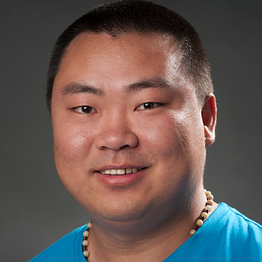# Everyone Can Understand Machine Learning — Regression Tree Model

## Introduction to the Regression Tree Model without formulas, equations, and scientific statements

This article is meant to explain the regression tree machine learning model without any buzzwords and scientific expressions, so you don’t need any pre-requisite knowledge or a Computer Science/Math degree to understand it.

As one of the most commonly used machine learning models, a decision tree is usually used for classification purposes. …

# Why Python Needs “pass” Statement

## When to use “pass”? Are there any alternatives?

For anyone like me switched to Python programming language from other languages, it is quite common to find that Python has some statements that are very unique. The “pass” statement is one of them, which you rarely can find such a thing in other programming languages. I have been using Java, C, C#, JavaScript, Objective-C, Swift, Scala and so on, none of them has the “pass” statement.

In fact, the “pass” statement is a very simple expression in Python. In this article, I’m going to introduce the several different aspects of this unique statement including its usage, the scenarios that need to use it, the benefit of using it, the reason that Python needs it and finally the potential alternatives. …

# Math for Programmers — Considerations From Decimal to Binary

## Why computers use the binary number system?

It is well known that binary numbers are the basis of the classic computer world that is built by John von Neumann. Yes, when I say the classic computer, it is the device you’re using at the moment unless you’re working as a researcher in the quantum computing area.

What are the considerations between the two different number systems? And why binary is used but not decimal? It is not difficult but important as the basic knowledge of computer science. In this article, I’ll share some considerations about decimal and binary number systems.

# The Decimal Number System

I still remember when I was a kid, the teacher starts to teach us some numbers larger than 10. When she mentioned 11, I wrote down “101” on my paper and thought that would be correct. Unlike “ten” and “one” that is different from “eleven” in English, in my mother tongue, the pronunciation of 10 followed by the pronunciation of 1 is exactly how to say 11. …